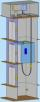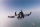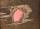# Flywheel

Flywheel turns 450 rev/min (RPM). Determine the magnitude of the normal acceleration of the flywheel point which are at a distance of 10 cm from the rotation axis.

Result

g =  222.07 m/s2

#### Solution:Leave us a comment of example and its solution (i.e. if it is still somewhat unclear...):Be the first to comment!## Next similar examples:

1. ElevatorIn homes with more floor elevators are used. For passenger transport, the most commonly used traction elevator counterweight. The top of the shaft engine room with the engine. The car is suspended on a rope, which is guided up over two pulleys to the count
2. Circular motionMass point moves moves uniformly in a circle with radius r = 3.4 m angular velocity ω = 3.6 rad/s. Calculate the period, frequency, and the centripetal acceleration of this movement.
3. BomberThe aircraft flies at an altitude of 4100 m above the ground at speed 777 km/h. At what horizontal distance from the point B should be release any body from the aircraft body to fall into point B? (g = 9.81 m/s2)
4. G forcesCalculate deceleration of car (as multiple of gravitational acceleration g = 9.81 m/s2) which occurs when a car in a frontal collision slows down uniformly from a speed 111 km/h to 0 km/h in 1.2 meters trajectory.
5. Free fallHow long does the stone fall freely into a depth of 80m? What speed will it hit the bottom of the abyss?
6. Free fallThe free fall body has gone 10m in the last 0.5s. Find the body speed at the moment of impact.
7. GravitationFrom the top of the 80m high tower, the body is thrown horizontally with an initial speed of 15 m/s. At what time and at what distance from the foot of the tower does the body hit the horizontal surface of the Earth? (use g = 10 ms-2)
8. ShotShot with a mass 43 g flying at 256 m/s penetrates into the wood to a depth 25 cm. What is the average force of resistance of wood?
9. AbyssStone was pushed into the abyss: 2 seconds after we heard hitting the bottom. How deep is the abyss (neglecting air resistance)? (gravitational acceleration g = 9.81 m/s2 and the speed of sound in air v = 343 m/s)
10. Pre účinnosťPre účinnosť bŕzd osobného vozidla je predpísané, že automobil pohybujúci sa po vodorovnej vozovke rýchlosťou 40km. H musí zastaviť na dráhe 15,4 m. Aké veľké je pritom spomalenie automobilu?
11. Train 2The train slowed down from 90 km/h to 72 km/h in 5 seconds. How long track travel?
12. CollisionThe two bodies, whose initial distance is 240 m, move evenly against each other consistently. The first body has an initial velocity of 4 m/s and an acceleration of 3 m/s2, the second body has an initial speed of 6 m/s and an acceleration of 2 m/s2. FindWhat overload in g (g-force) has passed the pilot if he accelerated from 0 to 600 km/h in 3 seconds?The driver of the car at a speed of 100 km/h faced the obstacle and began to brake with a slowing of 5 m/s². What is the path to stopping the car when the driver has registered the obstacle with a delay of 0.7 s?The car accelerates at rate 0.5m/s2. How long travels 400 meters and what will be its speed?We want to prove the sentence: If the natural number n is divisible by six, then n is divisible by three. From what assumption we started?Calculate the wavelength of the tone frequency 11 kHz if the sound travels at speeds of 343 m/s.